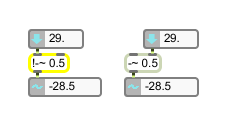# !-~

Signal subtraction (inlets reversed)

## Description

The !-~ object functions just like the -~ object, but the inlet order is reversed.

## Arguments

Name Type Opt Description
initial-subtraction-value float or int opt Sets an initial amount to subtract from the signal coming into the right inlet. If a signal is connected to the left inlet, the argument is ignored. If no argument is present, and no signal is connected to the left inlet, the initial value is 0 by default.

## Messages

 int input [int] In left inlet: An amount to subtract from the signal coming into the right inlet. If a signal is also connected to the left inlet, a float or int is ignored. In right inlet: Subtracts the signal coming into the left inlet from this value. If a signal is also connected to the right inlet, a float or int is ignored. float input [float] In left inlet: An amount to subtract from the signal coming into the right inlet. If a signal is also connected to the left inlet, a float or int is ignored. In right inlet: Subtracts the signal coming into the left inlet from this value. If a signal is also connected to the right inlet, a float or int is ignored. signal In left inlet: The signal is subtracted from the signal coming into the right inlet, or a constant value received in the right inlet. In right inlet: The signal coming into the left inlet or a constant value received in the left inlet is subtracted from this signal.

## Output

signal: The difference between the two inputs.

## Examples-~ with the inlets reversed